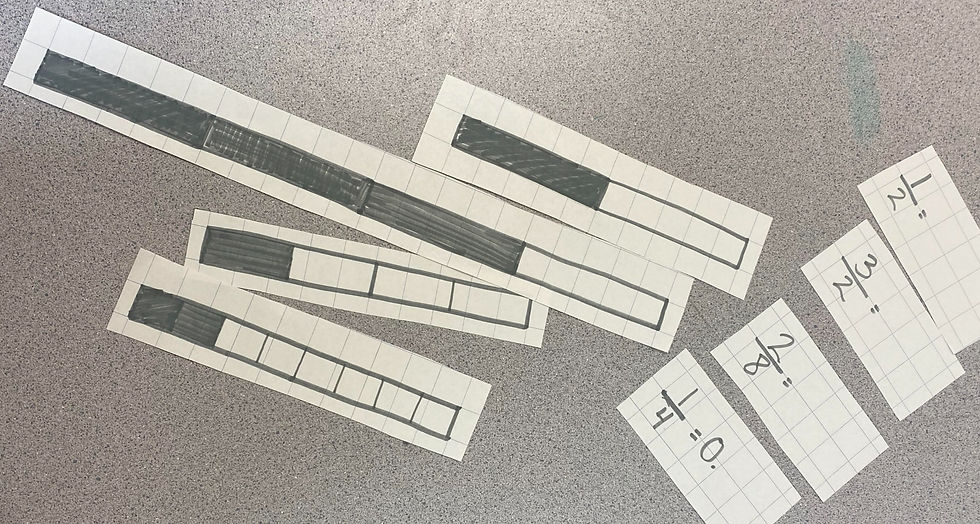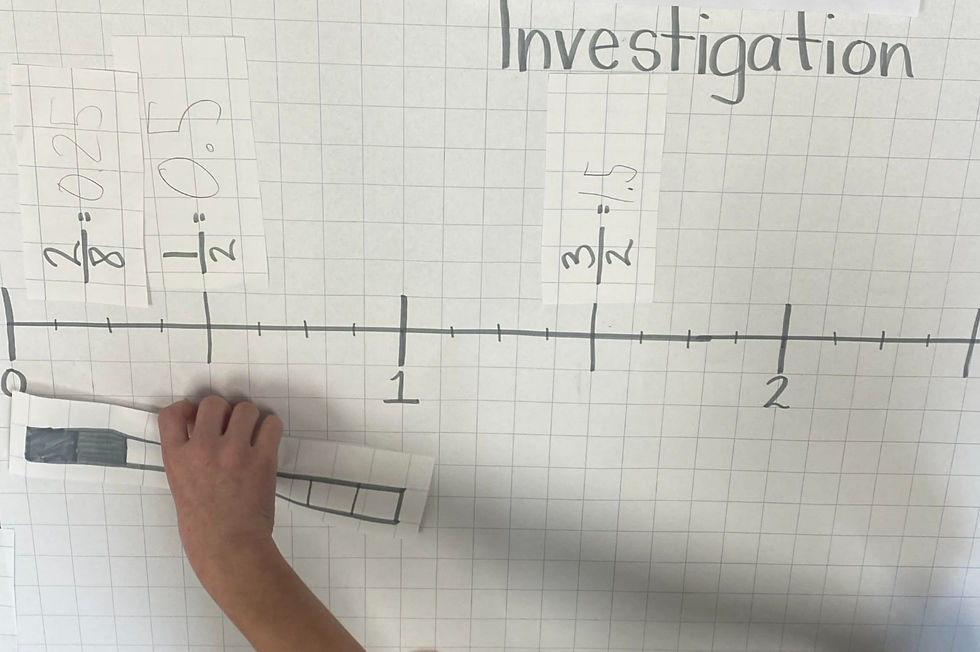top of page
Search

# Fractions, Decimals, and Percents 4/5

As we continue our min-series on fractions, today we are going to look at how fractions relate to decimals and percents.

First, let's take a look at the Ontario Curriculum to see when and how students will be asked to explore these skills at school:1. Explore the relationship between fractions and decimals

Starting in grade 4, students will begin to explore the relationship between fractions and decimals. In grade 4, this looks like noticing the relationship between 1/10 and 0.1 and 2/10 and 0.2. In grade 5, this looks like noticing the relationship between 1/4 and 0.25, the relationship between 1/100 and 0.01, and the relationship between 4/5, 80/100, and 0.8. In grade 6, this looks like noticing the relationship between 1/8 and 0.175, the relationship between 8/5 and 1.6, and the relationship between 0.625, 125/200, 25/40, and 5/8.

As you can see, the skill starts simple and builds over the three grades.

Home Connection Activity

Create a table and use a calculator to explore the relationship between 1/10, 2/10, 3/10, 4/10, 5/10, 6/10, 7/10, 8/10, 9/10, and 10/10 and their equivalent decimal numbers. What patterns appear?

2. Explore the relationship between fractions, decimals, and percents

Starting in grade 5, students are asked to explore the relationship between fractions, decimals and percents.

To convert a decimal number to a percent you multiply the decimal number by 100.

Home Connection Activity

Using the same table, create an additional column for percents. It is important to notice the relationship between the tenths and hundredths place value and the percentage. This will help alleviate errors when converting.

3. Convert between fractions, decimals, and percents

Converting between fractions, decimals, and percents is the act of dividing the numerator by the denominator.

Typically we convert fractions into decimals, and then decimals into percents. When converting decimals into percents, we want to align the numbers correctly. For example, 0.5 is 50% and 0.05 is 5%.

Here is a fun way that I introduced these skills

For this activity, we used calculators because we were first exploring how fractions can be represented as decimals.Converting decimals into fractions

When we have a decimal number, we need to know our place value amounts in order to successfully convert the decimal into a fraction.

For example:

0.1

The 1 is in the tenths column so the denominator is 10 and the numerator is 1.

Therefore 0.1 = 1/10

Example 2:

0.25

The 3 is in the hundredths column so the denominator is 100 and the numerator is 25.

Therefore 0.25 = 25/100

We can then simplify the fraction by dividing the numerator and denominator by the same number.

25/25 = 1

100/25=4

Therefore 0.25 = 25/100 = 1/4

A solid understanding of place value and fractions is critical for success with converting between fractions, decimals, and percents. Oftentimes, when students start to experience difficulty with these skills there are underlying foundational misconceptions.